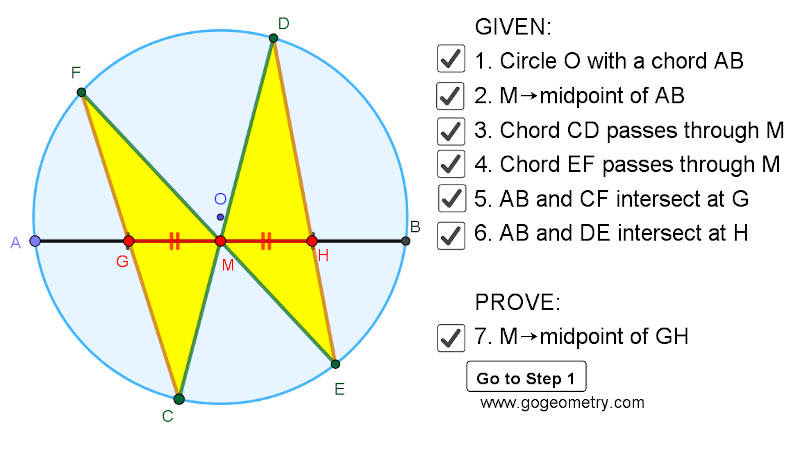## Saturday, April 25, 2020

### Dynamic Geometry 1474: Butterfly Theorem, Circle, Chords, Midpoints, Step-by-step Illustration

Interactive step-by-step animation using GeoGebra. Post your solution in the comment box below.
Level: Mathematics Education, High School, Honors Geometry, College.

Details: Click on the figure below.1.Draw HP // CF. From congrunce of two tr GM = MH
Full explanation will be in my sketch

1.https://photos.app.goo.gl/Vy6vC4caQVLi7Yat9

2.Draw perpendiculars OK,ON from O to CF,ED respectively

Obviously K and N are the midpoints of CF and ED respectively

Since Tr.s CFM and EDM are similar, CF/CM = ED/EM
Hence CK/CM = EN/EM & since < KCM = < MEN, Tr.s CKM & ENM are similar and so
< GKM = < MNE...….(1)

Now OMGK and OMNH are both concyclic so
< GKM = < GOM ….(2) and
< HOM = < MNE .…(3)

From (1), (2) & (3) we have < GOM = < HOM and hence GM = MH

Sumith Peiris
Moratuwa
Sri Lanka